# SBI PO Mains Reasoning (Day-02)

Dear Aspirants, Our IBPS Guide team is providing new series of Reasoning Questions for SBI PO 2021 Prelims so the aspirants can practice it on a daily basis. These questions are framed by our skilled experts after understanding your needs thoroughly. Aspirants can practice these new series questions daily to familiarize with the exact exam pattern and make your preparation effective.

Start Quiz

Puzzle

Direction (1-4): Read the given information carefully and answer the following questions.

There are 5 buildings A, B, C, D, and E such that building A is to the west of building B, building B is to the west of building C and so on. Building A has four floors, building B has three floors, building C has five floors, building D has two floors and building E has 4 floors. The bottommost floor is numbered 1, the floor above it is numbered 2 and so on.

Number of persons on each building from the bottommost floor to topmost floor is given as follows.

Building A: 51, 21, 15, 30

Building B: 40, 18, 34

Building C: 32, 21, 26, 17, 13

Building D: 19, 33

Building E: 28, 34, 42, 23

A dice throwing game is being played and 3 dices are thrown. As per the given conditions, the movements of persons take place.

I)If a prime number appears on the third dice and an even number appears on at least one of the other two dices, then the persons on the third floor of the building that is immediately next in east direction to the building with least number of floors moves to the third floor of the building that is immediately next in the west direction to the building having a maximum number of floors.

II)If a prime number appears on more than one of the dices, and an even number appears on the other dice then half the number of persons on the first floor of building E is moved to the second floor of the same building and then one – fourth of the total persons in the second-floor of that building moves to the third floor of building A and then one – third of the persons on the third floor of building A moves to the first floor of building C.

III) If an odd prime number appears on either of the first or third dice and two non-prime numbers appear on the other two dices then the persons on the second floors of all the first four buildings from west to the east together are equally distributed to the first three buildings from west to east. The persons move in such a way that the persons distributed to building A move to the first floor of building A, the persons distributed to building B move to the second floor of building B, and the remaining to the third floor of building C.

(Note: Take 1 as a non-prime number)

1) What will be the total number of persons in the buildings B and C together if 4, 2, and 5 appear on throwing the three dices respectively?

A.263

B.265

C.273

D.241

E. data insufficient

2) What will be the total number of persons in the buildings A, C, and E together if 3, 2, and 6 appear on throwing the three dices respectively?

A.340

B.353

C.343

D.334

E. data insufficient

3) On throwing the three dices, 5, 1, and 4 appear respectively. Then the number of persons on the even-numbered floors of all the buildings together is _____?

A.125

B.146

C.135

D.153

4) On throwing the three dices, different numbers appear on each dice and then a certain number of persons have moved from one building to another building accordingly. If the number of persons on the buildings B and E is 134, 85 respectively, then which of the following represents the outcomes of three dices up on throwing?

A.8, 2, and 3

B.5, 1, and 3

C.1, 4, and 3

D.6, 2, and 5

E.None of these

Critical reasoning

Direction (5):  A statement is given followed by six statements that speak further on the same theme and context. Read the statements and answer the questions that follow:

5) The stunning majority by which the National Democratic Alliance (NDA) government retained power in the 2019 general election is indeed a massive achievement. A host of factors have been responsible for this overwhelming majority, with issues relating to the political economy also contributing to this landslide.

i). The government made serious efforts towards several welfare schemes.

ii). Income support scheme provided direct income support of Rs. 6,000 per year to farmers who have up to 2 hectares of land.

iii). Socially and financially weaker sections of society did benefit from one scheme or the other.

iv). Major strides have been made such as green and white revolution, the agrarian crisis has always been a serious cause of concern

v). The ambit of PM-Kisan which was extended to all farmers which meant that an additional 20 million farmers.

vi). India’s agricultural sector has gone through massive reforms with both input and output subsidies being an important part of the government budget.

Which of the following largely contributed to the overwhelming majority?

A.1, 3 and 5

B.5, 2, 4 and 6

C.2, 5 and 6

D.1, 2 and 5

E.All of these

Blood relation- coded

Direction (6-10): Study the following information carefully and answer the questions given below it.

‘A & B’ means ‘A is the mother of B’.

‘A % B’ means ‘A is the father of B’.

‘A # B’ means ‘A is the sister of B’.

‘A @ B’ means ‘A is the brother of B’.

‘A ^ B’ means ‘A is the son of B’.

‘A * B’ means ‘A is the daughter of B’.

6) In the given expression below which of the following statement is not true?

N @ J & B # D ; K @ G & U; D % R # T ^ M; L * U @ M * F;

A.B is theaunty of T.

B.D isthe son-in-law of G.

C.R is thedaughter-in-law of F.

D.M is theniece of K.

E.All the given statements are true.

7) How H is related to U in the expression given below?

M @ H & W * G % T & U ^ L

A.Father-in-law

B.Maternal Grandmother

C.Sister-in-law

D.Maternal Aunty

E.None of these

8) In which of the following expression M is maternal Uncle of H?

A.D ^ R # W % M @ K % L @ H * U

B.R * D % M # W @ K & L @ H ^ U

C.W @ R & D  # W @ M %  K # H ^ U

D.R # D @ W & M @ K & L # H ^ U

E.None of these

9) Which of the following option correctly fill the blanks respectivelyso that D is thefather-in-law of H?

F # D__U__T * R__B % K__H

A.&, @, %, *

B.#, %, @, *

C.%, @, &, ^

D.&, *, %, ^

E.None of these

10) How U is related to V in the given expression given below?

R % L ^ U # K @ W & H # V

A.Mother-in-law

B.Maternal Aunty

C.Father-in-law

D.Grand Father

E.None of these

Directions (1-4):

From the given information, the diagrammatic representation of the buildings, its floors, and the number of persons on each floor are as follows.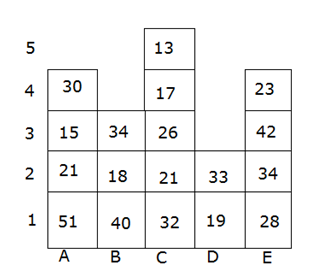The dices show 4, 2, and 5 respectively.

Here the third dice shows a prime number and the other two dices shows even numbers. So condition I may apply.

But 2 is an even prime number here. So condition II may also apply.

So, the data is not sufficient enough to answer the question.

On throwing the dices, they show 3, 2, and 6 respectively.

Here 2 and 3 are prime numbers and 6 is an even number. So condition II applies here.

As per condition II, half the number of persons on the first floor of the building E i.e. 14 persons moves to the second floor of the same building. So, the number of persons on the second floor of building E will be 34 + 14 = 48.

Now, one – fourth of the persons on the second floor of the building E i.e. 12 persons have to be moved to the third floor of building A. Then the number of persons on the third floor of building A will be 15 + 12 = 27.

Now, one – third of the number of persons on the third floor of building A i.e. 9 persons have to be moved to the first floor of building C. Then the number of persons on the first floor of building C will be 32 + 9 = 41.

After following all the steps, the number of persons on different floors of different buildings is as follows.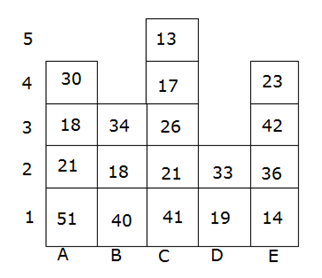The total number of persons in buildings A, C, and E =120 + 118 + 115= 353

The first dice shows a prime number and the other two dices are non-prime numbers. So, condition iii applies here.

As per condition III, the persons on the second floors of the first four buildings together i.e. 93 has to be equally distributed to the buildings A, B, and C. So 31 persons will be added to each building.

It is also given that they move in such a way that persons distributed to building A move to the first floor of building A, persons distributed to building B move to the second floor of building B, and the remaining to the third floor of building C.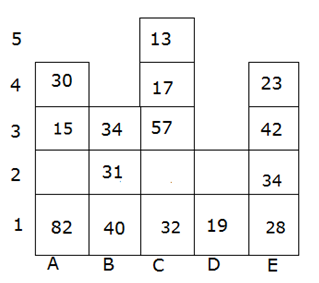The number of persons on the even-numbered floors of all the buildings together = 31 + 34 +30 +17 + 23 = 135

If we can observe clearly, the persons from the third floor of building E have moved to build B, which means the condition I is applied here.

So, the number on the third dice must be a prime number and any of the other two must be an even number. Option b can be eliminated for the same reason.

In option a and option d, there is an even prime number i.e.2. None of them could be the outcomes because if they are the outcomes, then it would make a clash between condition I and condition II. The data is insufficient in their case.

So, option c is correct.

The given statement is talking about the victory of NDA government which retained power in 2019 general elections. All the statements are talking about the efforts, schemes and policies made by the government for the welfare of the people. Therefore ‘all of these’ is the correct answer as all the statements are the facts that largely contributed to the overwhelming majority.

Directions (6-10):

We have:

N @ J & B # D; K @ G & U; D % R #  T ^ M; L *U @ M* F;

From the above given expression we have: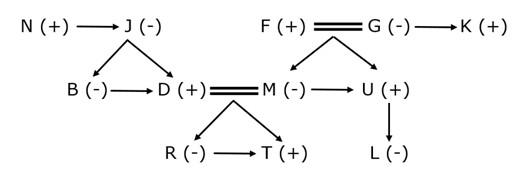Hence, option C is the correct choice.

We have:

M @ H & W * G % T & U ^ L

From theabove given expression we have: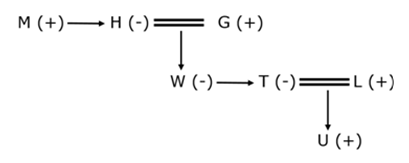Clearly, H is the maternal grandmother of U.

Hence, option B is the correct choice.

We have:

From option D we have: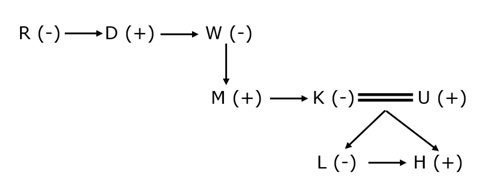Hence, option D is the correct choice.

From option C we have: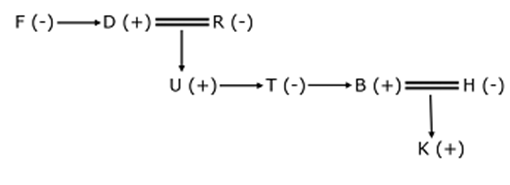Clearly, D is the father-in-law of H.

Hence, option C is the correct choice.

We have:

R % L ^  U  # K @ W & H # V

From the above given expression we have: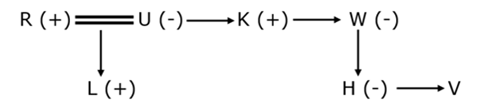Clearly, U is the maternal aunty of V.

Hence, option B is the correct choice.

1 1 vote
Rating